﻿ Electric Charging/Discharging Characteristics of Capacitor, Using De-alloyed Si-20Al Alloy RibbonsElectrical and Electronic Engineering

p-ISSN: 2162-9455    e-ISSN: 2162-8459

2013;  3(2): 72-76

doi:10.5923/j.eee.20130302.05

### Electric Charging/Discharging Characteristics of Capacitor, Using De-alloyed Si-20Al Alloy Ribbons

M. Fukuhara1, 2

1Research Institute for Electromagnetic Materials, Sendai, 982-0807, Japan

2Fracture & Reliability Research Institute, Tohoku University, Sendai, 980-8579, Japan

Correspondence to: M. Fukuhara, Research Institute for Electromagnetic Materials, Sendai, 982-0807, Japan.
 Email: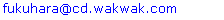Abstract

Temperature and voltage dependencies of capacitance of de-alloyed Si-20 at%. Al alloy ribbons were measured as a function of frequency between 1m Hz and 100 kHz, using exponential transient analysis for electric charging/discharging. In sharp contrast to conventional electric double layer capacitor (EDLC), the capacitance of the specimen obtained by prompt (below 0.1 s) charging/discharging has not substantially changed in temperature region from 193 to 423 K and voltage variation from 10 to 150 V, showing direct electric storage without solvents. The capacitance charging/discharging efficiency ratios is almost the same as 97 % in temperature region from 193 to 423 K and voltage variation from 10 from 150 V. Thus the de-alloyed alloy can be regarded as a “dry” capacitance cell composed of distributed constant equivalent circuits.

Keywords: De-Alloyed Si-Al, Electric Charging/Discharging, Temperature Dependence, Voltage Dependence, Electric-Distributed Constant Capacitor

Cite this paper: M. Fukuhara, Electric Charging/Discharging Characteristics of Capacitor, Using De-alloyed Si-20Al Alloy Ribbons, Electrical and Electronic Engineering, Vol. 3 No. 2, 2013, pp. 72-76. doi: 10.5923/j.eee.20130302.05.

### 1. Introduction

Electric storage is one of the topics currently attracting a great deal of interest in the field of energy source technology[1-6]. It can be accomplished by electrochemical devices and physical media. Much attention has been devoted to electrochemical energy storage for future electronic devices and electric power application, and storage of renewable energy for the power grid[7, 8].
Recently, we have found a (Ni0.36Nb0.24Zr0.40)90H10 glassy alloy, showing Coulomb dot oscillation at room temperature and a semi-true circular Nyquist diagram with total capacitance of 17.8 µF, derived from a large number of 0.23-nm-capacitors with femtofarad capacitance among its distorted icosahedral Zr5Ni5Nb3 clusters (dots of ca. 0.55 nm in size[11, 12]). However, it was difficult for the (Ni0.36Nb0.24Zr0.40)90H10 glassy alloy to discharge promptly discharging, because of a polarized glutinous liquid that is absorbed between pairs of metallic clusters.
In previous paper, with a view to promoting the prompt discharging, we have reported that de-alloyed crystalline Si1–xAlx (x = 0.2, 0.3, and 0.4) alloy ribbons with resistivities of 1–1000 Ωcm showed charging/discharging properties of 105 µF (0.55 F/cm3) during prompt period of 0.1 s at 1 mHz near dc current. Si grains were surrounded by many nanometer-sized canyons which are leached out by HCl acid treatment. It was considered that electric charge is stored into large numbers of canyons. From the observed electrode distance dependence on capacitance and nonlinear electronic transport behavior, we deduced that the alloy consisted of an electric distributed constant equivalent circuit (series with 1.7 % parallel), analogous to conventional electric double layer capacitors (EDLC)[15, 16]. Since the I-V characteristics showed Schottky junction, we found that the Si skeleton and Al backbone are to an electron what active carbon is to the electrolyte solution in EDLC.
In this study, we report temperature and voltage dependences of charging/discharging characteristics for the de-alloyed Si-20 at.% Al alloy ribbons with electric resistivity of 3 kΩcm. Si with forbidden band of 1.1 eV at room temperature is not dielectrics, which is characterized by forbidden band over about 2.5 eV, but semiconductor. Storage of electric charge in metals and alloys has to been overlooked in the literature, as far as we know. If we can store electricity in ac current, we can propose disuse of power transmission lines.

### 2. Experimental

The rotating wheel method under an argon atmosphere was used for preparing Si-20at%.Al alloy ribbons of 1 mm width and a thickness of about 50 µm. using rotating speed of 31.4 m/s. De-alloying of the samples was carried out for 259.2 ks in 1N HCl solution at room temperature. The details of the procedure have been described in previous paper.
Capacitances were calculated as a function of frequency between 1m Hz and 100 kHz from electric charge/discharge pulse curves of 10-150 V applied at 25 ns~0.1 s intervals, using a mixed-signal oscilloscope (MSO 5104, Tektronix) and 30 MHz multifunction generator (WF1973, NF Co.) on the basis of a simple exponential transient analysis. The schematic diagram for measurement of ribbon specimen is shown in Fig. 1 a. The distance between the two electrodes was 2 mm. Two gold wires each having a diameter of 100 µm were soldered to the alloy ribbon. We used an isothermal furnace in the temperature region from 193 to 423 K in air at ambient pressure. The charging/discharging efficient ratio was calculated from integration of transient curves for charging/discharging.

### 3. Results and Discussion

#### 3.1. Temperature and Voltage Dependent Capacitance

Since the de-alloyed Si-20 at.% Al is composed of distributed constant equivalent circuits of series C with 1.7 %parallel C, we cannot use the LCR meter which are measured on the basis of the parallel or series We measured the voltage transient phenomena as a function of time for charging and discharging in input and output circuits. The representative results for 1 mHz at 100V are presented at Figs. 1(b) and (c). Transient analysis of Figs. 1(b) and (c) gave capacitance values of 92.0 and 93.5 μF for charging and discharging, respectively. Since both values are almost the same, the capacitance value for discharging hereafter serves conveniently as capacitance one.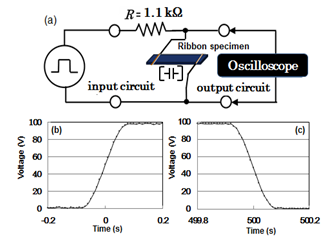Figure 1. A sample line graph using colors which contrast well both on screen and on a black-and-white hard copy
We first measured the temperature dependence of capacitance in the temperature region from 193 to 423 K at 10V. The results at 0.001, 0.01, 0.1, 1, 10, and 100 Hz are semi-logarithmically shown in Fig. 2(a). The capacitance for all the frequencies is apparently almost the same independent of temperature. Strictly speaking, the capacitance decreases 3 % at 193 K and 5 % at 423 K for room temperature value, as shown in Fig. 2(b) at 1 mHz. As regards the causes of slight deterioration, there is a possibility that decreases in lower and higher temperatures are derived from partial disappearance of parallel circuit due to shrinkage and increase in canyon size in the structure, respectively.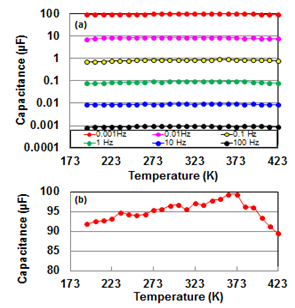Figure 2. (a) The temperature dependence of capacitance at 0.001, 0.01, 0.1, 1, 10, and 100 Hz in the temperature region from 193 to 423 K; (b) the temperature dependence of capacitance at 1 mHz in the temperature region from 193 to 423 K
However, we cannot make any assignment at present time. It needs further investigation.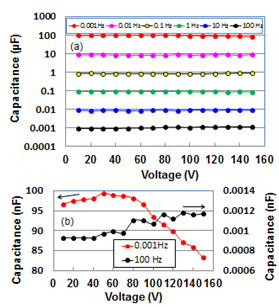Figure 3. (a) The voltage dependence of capacitance at 0.001, 0.01, 0.1, 1, 10, and 100 Hz in the voltage region from 10 to 150 V; (b) the temperature dependence of capacitance at 1 mHz in the temperature region from 10 to 150 V
We then measured voltage dependence of capacitance for frequency at room temperature. The result is semi-logarithmically shown in Fig. 3. We cannot see distinct capacitance change for voltage in the frequency region from 1 mHz to 100 Hz. Capacitance (Fig. 3(b)) at 1 mHz and 100 Hz in an equal axis show gradual decrease (15.2% at 150 V) from 80V to 150 V, and whole increase from 10 V, respectively. Judging from the whole increase at 100 Hz, the decrease from 80 V at 1 mHz would be derived from an electrostriction effect which is proportional to the square of the electric field for inhomogeneous materials in lower frequency region, although Si crystal does not show feroelectricity. The whole increase can be explained by a linear relation between dielectric polarization P and electric field E in paraelectrics, i.e., PE, where α is polarizability. The critical voltage which occurs self-discharging will be described in the following paper.

#### 3.2. Frequency Dependent Capacitance

Capacitances as a function of frequency for temperature and voltage are presented logarithmically in Fig. 4(a) and (b), respectively. Temperature dependent capacitances decreased parabolic from around 0.1 mF (0.54 F/cm3) to around 50 pF (0.3 µF/cm3) with increasing frequency and saturated in frequency region over 10 kHz. Here it should be noted that charging/discharging of electrochemical cells occurs at lower frequency regions on the whole interfaces in pores of electrodes, but does not occur at higher frequency ones in interior parts of pores. Hence, by analogy we infer that that the de-alloyed Si-Al alloy, which shows large frequency dependence on capacitance independent of temperature, is an assembly of canyons with the deepest recess.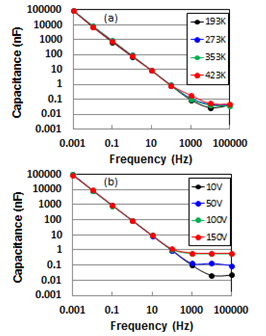Figure 4. Frequency dependence of capacitance for temperature at 193, 273, 353, and 423 K (a), and voltage at 10, 50, 100, and 150 V (b)
On the other hand, voltage dependent capacitances decreased parabolic up to 100 Hz, as well as the temperature dependent one, but capacitances at 100 and 150 V saturated in the frequency region over 100 Hz. The whole behaviours in Fig. 4 imply ac current momentary (below 0.1 s) charging/discharging, with the observed decrease in capacitance come from dielectric dispersion by interfacial polarization. These results would be associated with electron storage in Si-based solid cell without solvents. The de-alloyed Si-Al alloy of interest is completely different from the conventional “wet” cells such as EDLC and secondary cells which are controlled by diffusivity of ions. Thus, we can regard it as “dry” electric distributed constant capacitor (EDCC) with electric charging/discharging characteristics.
Furthermore, we can store electricity in ac current using a rectifier, if we could be taken a figure up four places over capacitance at higher frequencies.
Figure 5 shows a frequency dependent RC constant in input voltage of 10 V at room temperature. RC decreases parabolically from around 125 ms to around 30μs with increasing frequency up to 10 kHz at 100ms-15 ns intervals, before becoming saturated in the frequency region from 100 kHz to 1 MHz. The 125 ms at 1 mHz is 25 times larger than that (5 ms) in the conventional EDLC. However, it needs larger ones from 0.1 s to few hours for practical use.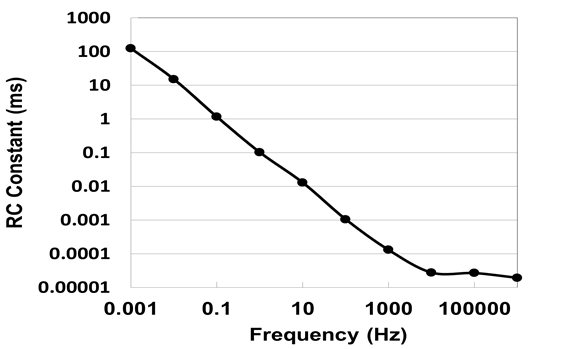Figure 5. Frequency dependence of RC constant in input voltage of 10 V at room temperature

#### 3.3. Temperature and Voltage Dependencies on Charging/Discharging Efficiency Ratio

Lastly, we analyze temperature and voltage dependencies on charging/discharging efficiency ratio. These results are shown at Fig. 6(a) and (b), respectively. Both efficiencies are almost the same as 97.0 % in temperature region from 193 to 423 K and voltage variation from 10 to 150 V. This means an inevitable leaking of 3 % for electric charge independent of temperature and voltage. Although the value is in quit agreement with that of EDLC at room temperature, the EDCC of interest shows superior performance in electrically storing at high temperatures and voltages, where EDLC cannot be used.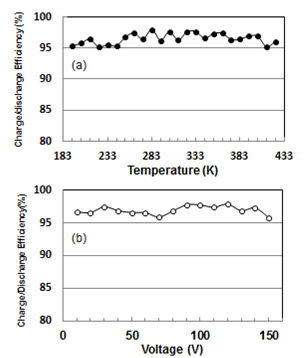Figure 6. Temperature (a) and voltage (b) dependencies on charg ing/discharging efficiency ratios at 1 mHz

### 4. Conclusions

Capacitance of de-alloyed crystalline Si-20 at.% Al alloy ribbon was investigated as functions of temperature and voltage in comparison with EDLC. The temperature dependent capacitances of the specimen decreased parabolic from around 100 µF (0.54 F/cm3) to around 50 pF (0.3 µF/cm3) with increasing frequency up to 10 kHz, before becoming saturated in the frequency region over 10 kHz. This indicates distinct ac current momentary (below 0.1 s) charging/discharging, showing electron storage in Si-based solid cell without solvents. The de-alloyed Si-Al alloy is completely different from the conventional “wet” cells such as EDLC and secondary cells which are controlled by diffusivity of ions. Therefore we can regard it as “dry” EDCC. The temperature and voltage dependent capacitances showed relatively small loss (3 %) in wide temperature between 193 and 423 K, and voltage region between 10 and 150 V. Thus the EDCC shows superior performance in electrically storing at high temperatures and voltages, where EDLC cannot be used.

### ACKNOWLEDGEMENTS

The author would like to thank Mr. T. Takahashi for the preparation of alloy ribbons. This work was supported by a Grant-in-Aid for Science Research in a Priority Area, ‘Advanced Low Carbon Technology Research and Development Program’, from the Japan Science and Technology, Japan.

### References

  M.Winter, R.J. Broard. 2004,“What are batteries, fuel cells, and supercapacitors?”, Chem. Rev., 104: 4245-4269.  M.S. Whittingham, 2008, “Materials Challenges Facing Electrical Energy Storage”, MRS Bull., 33, 411-419.  B.E.Conway, 1999, “Electrochemical Super-capacitors: Scientific Fundamentals and Technology Applications”, Plenum Press, New York.  P. Simon and Y. Gogotsi. 2008, “Materials for electrochemical capacitors”, Nat. Mat., 7, 845-854.  A.S. Arico, P. Bruce, B. Scrosativ, J.M.Tarascom and W.Schalkwijk, 2005, “Nanostructured materials for advanced energy conversion and storage devices”, Nat. Mat., 4, 366-377.  M. F. El-Kady. V. Strong, S. Dubin. R. B. Kaner, 2012, “Laser Scribing of High- Performance and Flexible Graphene-Based Electrochemical Capacitors”, Science. 335, 1326-1330.  J. R. Miller and P. Simon, 2008, “Electrochemical Capacitors for Energy Management”, Science, 321,651-652.  M. Armand and J. M. Tarascon, 2008, “Building better batteries”, Nature, 451, 652-657.  M.Fukuhara and A. Inoue, 2009, “Room-temperature Coulomb oscillation of a proton dot in Ni-Nb-Zr-H glassy alloys with nanofarad capacitance,” J. Appl. Phys.105, 063715.  M. Fukuhara, M. Seto and A.Inoue, 2010, “ac impedance analysis of a Ni-Nb-Zr-H glassy alloy with femtofarad capacitance tunnels”, Appl. Phys. Lett., 96, 043103.  M. Fukuhara, N. Fujima, H. Oji, A. Inoue and S. Emura, 2010, “Structures of the icosahedral clusters in Ni-Nb-Zr-H glassy alloys determined by first-principles molecular dynamics calculation and XAFS measurements”, J. Alloy. Comp.,497, 182-187.  M. Fukuhara, H. Yoshida, K. Koyama, A.Inoue A and Y. Miura, 2010, “Electronic transport behaviors of Ni-Nb-Zr-H glassy alloys”, J. Appl. Phys., 107, 033703.  M. Fukuhara, H.Yoshida, N.Fujima and H.Kawarada. 2012, “Capacitance Distribution of Ni-Nb-Zr-H Glassy Alloys”, J. Noanosci. Nanotech., 12, 3848-3852.  M.Fukuhara, T.Araki, K.Nagayama and H.Sakuraba, 2012, “Electric storage in de-alloyed Si-Al alloy ribbons”, Europhys. Lett., 99, 47001.  D. C. Grahame, 1947, “The Electrical Double Layer and the Theory of Electrocapillarity”, 41, 441-501.  M. Okamura, 2011, “Electric Double Layer Capacitor and Its Storage System”, Nikkan Kogyo, Tokyo.  H. Tsuji H. et al., 2004, “Silver nanoparticle formation in thin oxide layer on silicon by silver-negative-ion implantation for Coulomb blockade”, Appl. Surf. Sci., 238, 132-137.  T. Nakamura, 1989, “Feroelectricity involved in structural phase transitions”, Shokabo, Tokyo.  M. Itagaki, 2008, “Electrochemistry, Impedance Method”, Maruzen, Tokyo, p. 135.